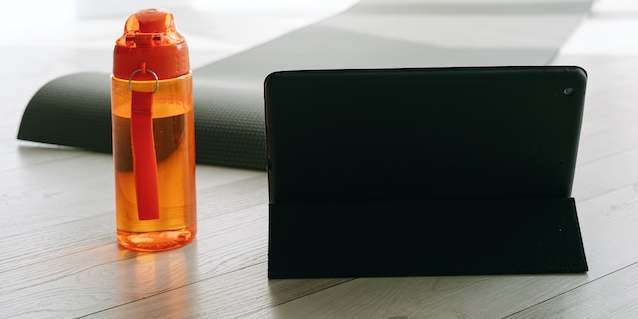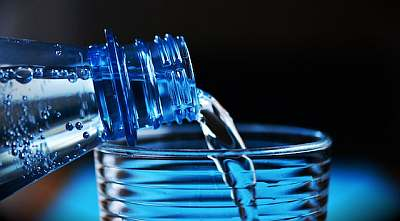# Ounces to Liters

Instructions: Use this calculator to convert ounces to liters, showing all the steps of the process. Please type the number of ounces you would like to convert in the form below:Type the number of ounces:

## Converting Ounces to Liters

This calculator is about converting ounces to liters. Also, using the exact same procedure, just solving for the other variable, you can easily convert from liters to ounces.

As you will see below, the process is completely straightforward, once you understand the rationale behind it.

So, as easy as the process is, and as you attempt lots of conversion of units, you will realize that is not completely easy to memorize all conversions, so the need for a conversion tool is clear..## How do you convert 1 ounce to liters?

For the ounces to liter conversion, we first, let's start off saying that 1 ounce is smaller than 1 liter, so we will revert the question to how many ounces in 1 liter?. The answer is simple: there are 33.814 ounces in a liter.

## How do you convert ounces to liters

Mathematically, the conversion is simple: You will need to divide the number of ounces you want to convert by a constant. The interesting part is understanding which constant is used for this purpose.

## What are the steps for the conversion of ounces into cups

• Step 1: Establish the initial equivalence. We know that 1 liter is the same as 33.814 ounces
• Step 2: Then, identify precise what is the amount of ounces (O) you want to convert
• Step 3: Now, we establish the basic proportion: $$\displaystyle \frac{33.814}{1} = \frac{O}{L}$$
• Step 4: Finally, solving the basic the proportion for L, we get the following conversion formula: $$L = \displaystyle \frac{O}{33.814}$$

## Why would you want to convert ounces to liters

Lots of people may not be aware that not every country uses the same volume units. Then, a conversion tool between ounces and liters can be super useful.

And considering that there are lots of different units you can convert back and forth (especially in the imperial units system), a conversion tool like this one can help you, when the necessity arises.### Example: Converting ounces to liters

Convert 10 ounces to liters

Solution: We first need to identify the volume that needs to be converted, which in this case is O = 10 ounces. Second, the conversion formula to be used is:

$L = \displaystyle \frac{O}{33.814}$

Then, we plug in O = 10 ounces into the above formula:

$L = \displaystyle \frac{O}{33.814} =\displaystyle \frac{10}{33.814} = 0.296 \text{ liters}$

### Example: Another conversion

Convert 64 ounces to liters

Solution: The conversion formula is

$L = \displaystyle \frac{O}{33.814}$

Then, we plug in O = 64 ounces into the above formula:

$L = \displaystyle \frac{O}{33.814} =\displaystyle \frac{64}{33.814} = 1.893 \text{ liters}$

### Example: Another ounces to liters conversion

Convert 2 liters to ounces

Solution: The conversion formula we need to use is:

$L = \displaystyle \frac{O}{33.814}$

But in this case we have that O is given instead of L, so we solve for O in the above equation, and we get

$L = \displaystyle \frac{O}{33.814} \Rightarrow O = 33.814 L$

So now, plugging L = 2 above:

$O = 33.814 L = 33.814 \times 2 = 67.628$

## Other useful volume units conversions

There are many conversions that you may be interested in such as doing cups to ounces and reversely, ounces to cups.

Typically, conversions within each unit system will be with round easy to remember numbers. Though numbers get more complicated when you are converting across unit systems, as a general rule.

Other conversion calculators of interest could a cups to quarts and quarts to gallons, among many others that you can try.